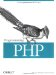3.7 Anonymous Functions

Some PHP functions use a function you provide them with to do part of their work. For example, the usort( ) function uses a function you create and pass to it as a parameter to determine the sort order of the items in an array.

Although you can define a function for such purposes, as shown previously, these functions tend to be localized and temporary. To reflect the transient nature of the callback, create and use an anonymous function (or lambda function).

You can create an anonymous function using create_function( ). This function takes two parameters the first describes the parameters the anonymous function takes in, and the second is the actual code. A randomly generated name for the function is returned:

\$func_name = create_function(args_string, code_string);

Example 3-7 shows an example using usort( ).

Example 3-7. Anonymous functions
\$lambda = create_function('\$a,\$b', 'return(strlen(\$a) - strlen(\$b));'); \$array = array('really long string here, boy', 'this', 'middling length', 'larger'); usort(\$array, \$lambda); print_r(\$array);

The array is sorted by usort( ), using the anonymous function, in order of string length.Programming PHP
ISBN: 1565926102
EAN: 2147483647
Year: 2007
Pages: 168

Similar book on Amazon